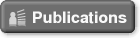### Carlos A. Coelho

 Carlos A. Coelho       (Carlos Agra Coelho)       (Carlos Manuel Agra Coelho)       Full Professor      Mathematics Department - Faculdade de Ciências e Tecnologia - Universidade Nova de Lisboa                              2829-516 Caparica, Portugal      Ph.D. in Biostatistics by The University of Michigan, Ann Arbor   Elected Member of the International Statistical Institute                     Office:      Phone: +351 21 2948388 (ext: 10855) Fax:     +351 21 2948394 E-mail:  cmac@fct.unl.pt             Research Interests: Mathematical Statistics - Multivariate Statistics Distribution Theory Near-exact Distributions, Asymptotic Distributions, Exact Distributions Computational StatisticsStatistical Disclosure Control Linear, Generalized Linear and Mixed Models (Univariate and Multivariate)Applied Statistics                   Editorial Positions                 Associate Editor:            Member of Editorial Board:Discussiones Mathematicae - Probability and Statistics           Publications              A list of the publications is available at Google Scholar (Carlos A. Coelho)  and also at(Publications list )                BooksCoelho, C. A., Arnold, B. A. (2019). Finite Form Representations for Meijer G and Fox H functions -- Applied to Multivariate Likelihood Ratio Tests Using Mathematica, MAXIMA and R, Lecture Notes in Statistics, Springer (ISBN: 978-3-030-28789-4)                                (The book has a supplementary and complementary material web-page)Coelho, C. A., Mexia, J. T. (2010) Product and Ratio of Generalized Gamma-Ratio Random Variables: Exact and Near-exact Distributions - Applications. Lambert Academic Publishing AG & Co. KG, Saarbrucken, Germany, 145+v pp.(ISBN: 978-3-8383-5846-8)              Papers            Accepted for publication:              Marques, F. J., Coelho, C. A. (2020). Testing simultaneously different covariance block diagonal structures – the multi-sample case. Journal of Applied Statistics (accepted for publication).            Recently published:Coelho, C. A., Roy, A. (2020). Testing the hypothesis of a doubly exchangeable covariance matrix. Metrika, 83, 45-68.Marques, F. J., Coelho, C. A. (2018). The simultaneous test of equality and circularity of several covariance matrices, Journal of Statistical Theory and Practice, 12, 861-885.Correia, B. R., Coelho, C. A., Marques, F. J. (2018). Likelihood ratio test for the hyper-block matrix sphericity covariance structure – characterization of the exact distribution and development of near-exact distributions for the test statistic, REVSTAT, 16, 365-403.Coelho, C. A. (2017). The likelihood Ratio Test for Equality of Mean Vectors with Compound Symmetric Covariance Matrices, in Computational Science and Its Applications, Lecture Notes in Computer Science 10408, Vol. V, Springer, pp. 20-32 (ISBN: 978-3-62403-7, 978-3-62404-4 (e-Book).Marques, F. J., Coelho, C. A., Rodrigues, P. C. (2017). Testing the equality of several linear regression models, Computational Statistics, 32, 1453-1480.Coelho, C. A., Roy, A. (2016). Testing the hypothesis of a block compound symmetric covariance matrix for elliptically contoured distributions, TEST, 26, 308-330.Moura, R., Klein, M., Coelho, C. A., Sinha, B. (2017). Inference for Multivariate Regression Model based on Synthetic Data generated under Fixed-Posterior Predictive Sampling: Comparison with Plug-in Sampling, REVSTAT, 15, 2, 155-186.Grilo, L. M., Coelho, C. A. (2017). The exact and near-exact distributions for the statistic used to test the reality of covariance matrix in a complex normal distribution, in Applied and Computational Matrix Analysis, Springer Proceedings in Mathematics & Statistics 192, pp. 295-315, Springer (ISBN: 978-3-319-49982-6).Kedem, B., Pan, L., Zhou, W., Coelho, C. A. (2016). Interval Estimation of Small Tail Probabilities – Applications in Food Safety, Statistics in Medicine, 35, 3229–3240.Marques, F. J., Coelho, C. A. (2016). Near-exact Distributions for Positive Linear Combinations of Independent Non-central Gamma Random Variables, AIP Conference Proceedings, 1738, 190005-1–190005-.4Coelho, C. A., Marques, F. J., Oliveira, S. (2016). Near-exact distributions for likelihood ratio statistics used in the simultaneous test of conditions on mean vectors and patterns of covariance matrices, Mathematical Problems in Engineering, Volume 2016, Article ID 8975902, 25 pages.Coelho, C. A., Marques, F. J., Arnold, B. C. (2015). The exact and near-exact distributions of the main likelihood ratio test statistics used in the complex multivariate normal setting, Test, 24, 386-416 (+supplementary material, 14pp).Marques, F. J., Coelho, C. A., de Carvalho, M. (2015) On the distribution of linear combinations of independent Gumbel random variables. Statistics and Computing, 25, 3, 683-701 (+supplementary material, 5pp)Marques, F. J., Coelho, C. A. (2015). Near-exact Distributions for the Likelihood Ratio Test Statistic for Testing Multi-Sample Independence The Real and Complex Cases, Journal of Statistical Theory and Practice, 9, 37-58.Coelho, C. A., Arnold, B. C. (2014) On the exact and near-exact distributions of the product of generalized Gamma random variables and the generalized variance. Communications in Statistics - Theory and Methods, 43, 2007-2033.